# ML | Logistic Regression using Tensorflow

Prerequisites: Understanding Logistic Regression and TensorFlow.

Brief Summary of Logistic Regression:
Logistic Regression is Classification algorithm commonly used in Machine Learning. It allows categorizing data into discrete classes by learning the relationship from a given set of labeled data. It learns a linear relationship from the given dataset and then introduces a non-linearity in the form of the Sigmoid function.

In case of Logistic regression, the hypothesis is the Sigmoid of a straight line, i.e,whereWhere the vector w represents the Weights and the scalar b represents the Bias of the model.
Let us visualize the Sigmoid Function –

 import numpy as np  import matplotlib.pyplot as plt     def sigmoid(z):      return 1 / (1 + np.exp( - z))     plt.plot(np.arange(-5, 5, 0.1), sigmoid(np.arange(-5, 5, 0.1)))  plt.title('Visualization of the Sigmoid Function')     plt.show()

Output: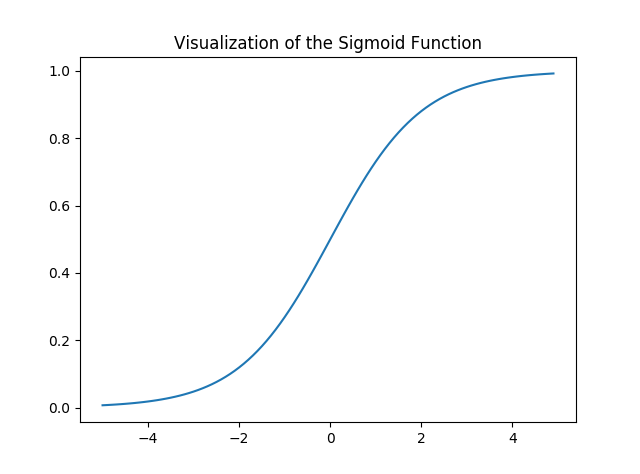Note that the range of the Sigmoid function is (0, 1) which means that the resultant values are in between 0 and 1. This property of Sigmoid function makes it a really good choice of Activation Function for Binary Classification. Also for z = 0, Sigmoid(z) = 0.5 which is the midpoint of the range of Sigmoid function.

Just like Linear Regression, we need to find the optimal values of w and b for which the cost function J is minimum. In this case, we will be using the Sigmoid Cross Entropy cost function which is given byThis cost function will then be optimized using Gradient Descent.

Implementation:
We will start by importing the necessary libraries. We will use Numpy along with Tensorflow for computations, Pandas for basic Data Analysis and Matplotlib for plotting. We will also be using the preprocessing module of Scikit-Learn for One Hot Encoding the data.

 # importing modules  import numpy as np  import pandas as pd  import tensorflow as tf  import matplotlib.pyplot as plt  from sklearn.preprocessing import OneHotEncoder

Next we will be importing the dataset. We will be using a subset of the famous Iris dataset.

 data = pd.read_csv('dataset.csv', header = None)  print("Data Shape:", data.shape)     print(data.head())

Output:

Data Shape: (100, 4)
0    1    2  3
0  0  5.1  3.5  1
1  1  4.9  3.0  1
2  2  4.7  3.2  1
3  3  4.6  3.1  1
4  4  5.0  3.6  1

Now let’s get the feature matrix and the corresponding labels and visualize.

 # Feature Matrix  x_orig = data.iloc[:, 1:-1].values     # Data labels  y_orig = data.iloc[:, -1:].values     print("Shape of Feature Matrix:", x_orig.shape)  print("Shape Label Vector:", y_orig.shape)

Output:

Shape of Feature Matrix: (100, 2)
Shape Label Vector: (100, 1)

Visualize the given data.

 # Positive Data Points  x_pos = np.array([x_orig[i] for i in range(len(x_orig))                                      if y_orig[i] == 1])     # Negative Data Points  x_neg = np.array([x_orig[i] for i in range(len(x_orig))                                      if y_orig[i] == 0])     # Plotting the Positive Data Points  plt.scatter(x_pos[:, 0], x_pos[:, 1], color = 'blue', label = 'Positive')     # Plotting the Negative Data Points  plt.scatter(x_neg[:, 0], x_neg[:, 1], color = 'red', label = 'Negative')     plt.xlabel('Feature 1')  plt.ylabel('Feature 2')  plt.title('Plot of given data')  plt.legend()     plt.show()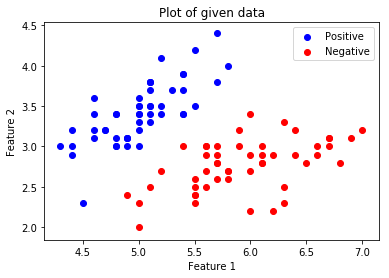.

Now we will be One Hot Encoding the data for it to work with the algorithm. One hot encoding transforms categorical features to a format that works better with classification and regression algorithms. We will also be setting the Learning Rate and the number of Epochs.

 # Creating the One Hot Encoder  oneHot = OneHotEncoder()     # Encoding x_orig  oneHot.fit(x_orig)  x = oneHot.transform(x_orig).toarray()     # Encoding y_orig  oneHot.fit(y_orig)  y = oneHot.transform(y_orig).toarray()     alpha, epochs = 0.0035, 500 m, n = x.shape  print('m =', m)  print('n =', n)  print('Learning Rate =', alpha)  print('Number of Epochs =', epochs)

Output:

m = 100
n = 7
Learning Rate = 0.0035
Number of Epochs = 500

Now we will start creating the model by defining the placeholders X and Y, so that we can feed our training examples x and y into the optimizer during the training process. We will also be creating the trainable Variables W and b which can be optimized by the Gradient Descent Optimizer.

 # There are n columns in the feature matrix  # after One Hot Encoding.  X = tf.placeholder(tf.float32, [None, n])     # Since this is a binary classification problem,  # Y can take only 2 values.  Y = tf.placeholder(tf.float32, [None, 2])     # Trainable Variable Weights  W = tf.Variable(tf.zeros([n, 2]))     # Trainable Variable Bias  b = tf.Variable(tf.zeros())

Now declare the Hypothesis, Cost function, Optimizer and Global Variables Initializer.

 # Hypothesis  Y_hat = tf.nn.sigmoid(tf.add(tf.matmul(X, W), b))     # Sigmoid Cross Entropy Cost Function  cost = tf.nn.sigmoid_cross_entropy_with_logits(                      logits = Y_hat, labels = Y)     # Gradient Descent Optimizer  optimizer = tf.train.GradientDescentOptimizer(           learning_rate = alpha).minimize(cost)     # Global Variables Initializer  init = tf.global_variables_initializer()

Begin the training process inside a Tensorflow Session.

 # Starting the Tensorflow Session  with tf.Session() as sess:             # Initializing the Variables      sess.run(init)             # Lists for storing the changing Cost and Accuracy in every Epoch      cost_history, accuracy_history = [], []             # Iterating through all the epochs      for epoch in range(epochs):          cost_per_epoch = 0                    # Running the Optimizer          sess.run(optimizer, feed_dict = {X : x, Y : y})                     # Calculating cost on current Epoch          c = sess.run(cost, feed_dict = {X : x, Y : y})                     # Calculating accuracy on current Epoch          correct_prediction = tf.equal(tf.argmax(Y_hat, 1),                                            tf.argmax(Y, 1))          accuracy = tf.reduce_mean(tf.cast(correct_prediction,                                                   tf.float32))                     # Storing Cost and Accuracy to the history          cost_history.append(sum(sum(c)))          accuracy_history.append(accuracy.eval({X : x, Y : y}) * 100)                     # Displaying result on current Epoch          if epoch % 100 == 0 and epoch != 0:              print("Epoch " + str(epoch) + " Cost: "                             + str(cost_history[-1]))             Weight = sess.run(W) # Optimized Weight      Bias = sess.run(b)   # Optimized Bias             # Final Accuracy      correct_prediction = tf.equal(tf.argmax(Y_hat, 1),                                        tf.argmax(Y, 1))      accuracy = tf.reduce_mean(tf.cast(correct_prediction,                                                tf.float32))      print("\nAccuracy:", accuracy_history[-1], "%")

Output:

Epoch 100 Cost: 125.700202942
Epoch 200 Cost: 120.647117615
Epoch 300 Cost: 118.151592255
Epoch 400 Cost: 116.549999237

Accuracy: 91.0000026226 %

Let’s plot the change of cost over the epochs.

 plt.plot(list(range(epochs)), cost_history)  plt.xlabel('Epochs')  plt.ylabel('Cost')  plt.title('Decrease in Cost with Epochs')     plt.show()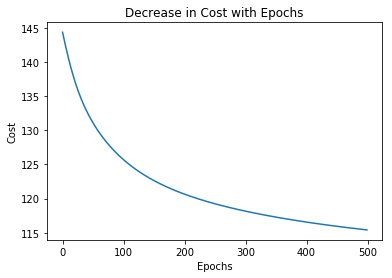Plot the change of accuracy over the epochs.

 plt.plot(list(range(epochs)), accuracy_history)  plt.xlabel('Epochs')  plt.ylabel('Accuracy')  plt.title('Increase in Accuracy with Epochs')     plt.show()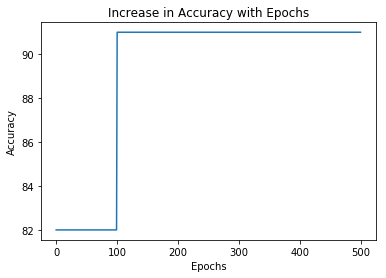Now we will be plotting the Decision Boundary for our trained classifier. A decision boundary is a hypersurface that partitions the underlying vector space into two sets, one for each class.

 # Calculating the Decision Boundary  decision_boundary_x = np.array([np.min(x_orig[:, 0]),                                 np.max(x_orig[:, 0])])     decision_boundary_y = (- 1.0 / Weight) *       (decision_boundary_x * Weight + Bias)     decision_boundary_y = [sum(decision_boundary_y[:, 0]),                          sum(decision_boundary_y[:, 1])]     # Positive Data Points  x_pos = np.array([x_orig[i] for i in range(len(x_orig))                                      if y_orig[i] == 1])     # Negative Data Points  x_neg = np.array([x_orig[i] for i in range(len(x_orig))                                       if y_orig[i] == 0])     # Plotting the Positive Data Points  plt.scatter(x_pos[:, 0], x_pos[:, 1],    color = 'blue', label = 'Positive')     # Plotting the Negative Data Points  plt.scatter(x_neg[:, 0], x_neg[:, 1],     color = 'red', label = 'Negative')     # Plotting the Decision Boundary  plt.plot(decision_boundary_x, decision_boundary_y)  plt.xlabel('Feature 1')  plt.ylabel('Feature 2')  plt.title('Plot of Decision Boundary')  plt.legend()     plt.show()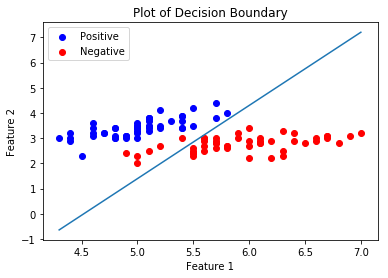My Personal Notes arrow_drop_upCheck out this Author's contributed articles.

If you like GeeksforGeeks and would like to contribute, you can also write an article using contribute.geeksforgeeks.org or mail your article to contribute@geeksforgeeks.org. See your article appearing on the GeeksforGeeks main page and help other Geeks.

Please Improve this article if you find anything incorrect by clicking on the "Improve Article" button below.

Improved By : nidhi_biet